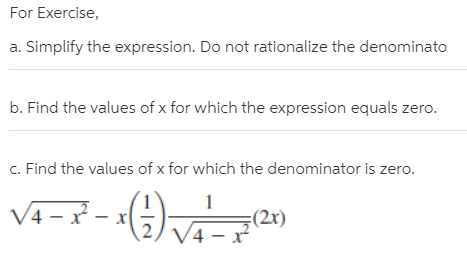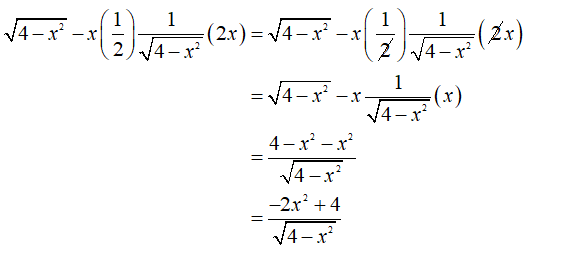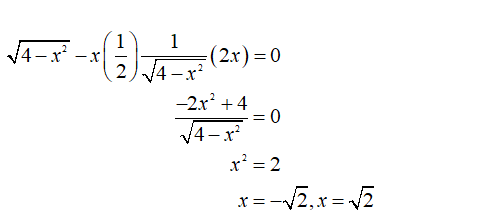# For Exercise,a. Simplify the expression. Do not rationalize the denominatob. Find the values of x for which the expression equals zero.c. Find the values of x for which the denominator is zero./4 – x -(2x)V4 – x

Question
1 viewshelp_outlineImage TranscriptioncloseFor Exercise, a. Simplify the expression. Do not rationalize the denominato b. Find the values of x for which the expression equals zero. c. Find the values of x for which the denominator is zero. /4 – x - (2x) V4 – x fullscreen
check_circle

Step 1

Part (a):

In the first step do not rationalize the denominator:Step 2

Part (b):

The value of x for which the above expression is zero:...

### Want to see the full answer?

See Solution

#### Want to see this answer and more?

Solutions are written by subject experts who are available 24/7. Questions are typically answered within 1 hour.*

See Solution
*Response times may vary by subject and question.
Tagged in